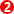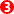﻿ Getting Started Tutorials - MechDesigner > Tutorial 16: Motion-Path FB and Blend-Curves > Step 16.1: About the Blend-Curve & Motion-Path FB

# Step 16.1: About the Blend-Curve & Motion-Path FB

### A Motion-Part and a Motion-Point

A Motion-Part and a Motion-Point are very similar elements:

Motion-Part: a Part, whose motion is constrained by a joint, whose position is identified by a Motion Dimension, and whose motion is defined by the input to the Motion-Dimension FB.

Motion-Point: a Point, whose motion is constrained by a sketch-path, whose position is identified by its Motion-Point Dimension, and whose motion is defined by the input to the Motion-Path FB.

Motion-Path FB & Motion-PointAdd Motion-Path FB: 1.Adds a Motion-Pointto a sketch-element/sketch-path AND specifies its position relative to the start-Point of the sketch-element. 2.Adds a Motion-Path FBto the graphic-area 3.Adds a dimension to the Motion-Pointto indicate how far the Motion-Point is from the start-Point of the sketch-element in 1. Note: To see the Motion-Point Dimensionyou must: 1.Edit the Motion-Path FB In the Motion-Path dialog-box > Point List and Data Display  separator: 2.Click the Displacement / Phase radio-button Displacement: the linear distance along the sketch-path. Phase: the distance along the sketch-path normalized between 0 and 360. 360 is the complete length of the sketch-path.

Motion-Dimension FB & Motion-PartAdd Motion-Dimension FB 1.Specifies the position of a sketch-element(Line or Point) in a Motion-Part relative to a different sketch-element (Line or Point) in another Part. 2.Adds a Motion-Dimension FBto the graphic-area. 3.Adds a Motion Dimensionto indicate the dimension between the sketch-elements in the two Parts. Motion-Part: a Part constrained by a joint. Its position, relative to a sketch-element in a different Part, is the total of values given by: Motion-values at the input-connector of the Motion-Dimension FB. Base-Value as entered in the Motion-Dimension dialog-box.

#### Why use a Blend-CurveA Blend-Curve is a sketch-element. Use the Blend-Curve dialog-box to specify, at its start-Pointand its end-Point, these parameters: •Angle •Curvature •Rate-of-Change of Curvature •Velocity-Scaling a normalization parameter that controls the overall length and shape of the Blend-Curve. Its advantage is its smoothness and how it blends with other sketch-elements at its start-point and end-Point. Question: Why do we need a Blend-Curve? Answer: To eliminate a curvature-discontinuity. Question: What is a curvature-discontinuity? Answer: A Curvature Discontinuity is a step change in Radius-of-Curvature. E.g. an Arc that is joined to a Line (and vice versa), or even an Arc to a an Arc with a different radii. Question: What is wrong with a curvature-discontinuity? Answer: A machine element that you join to a Motion-Point that moves along a sketch-path with the curvature-discontinuity will vibrate the machine-element. To reduce mechanical vibrations, you must eliminate the curvature-discontinuity. The purpose of the Blend-Curve element is to eliminate curvature-discontinuities as the Motion-Point moves along the sketch-path and between sketch-elements.